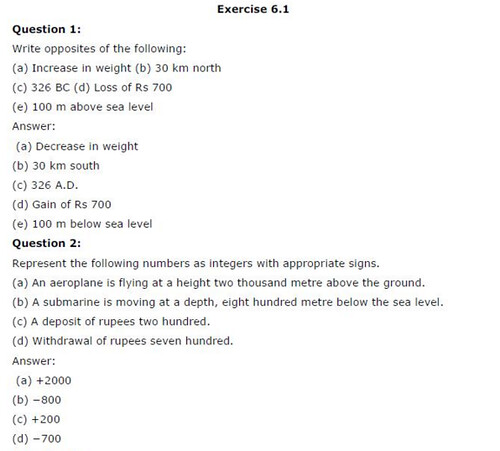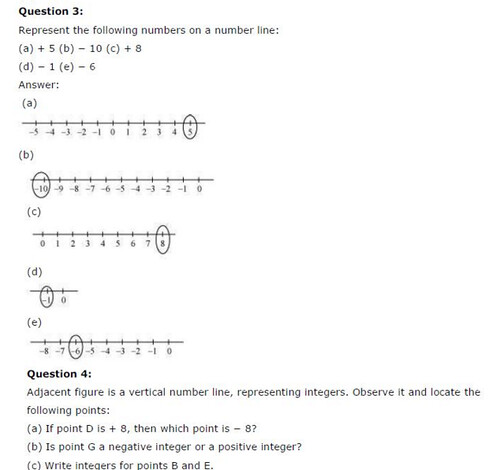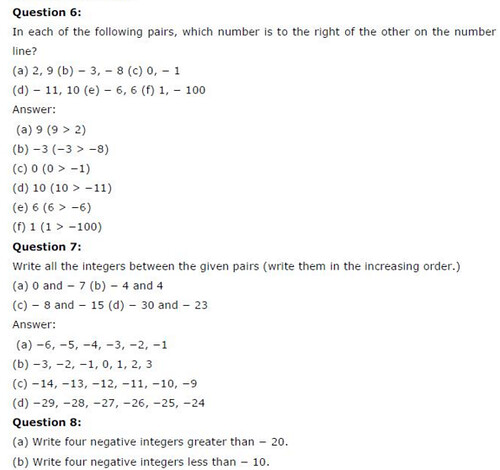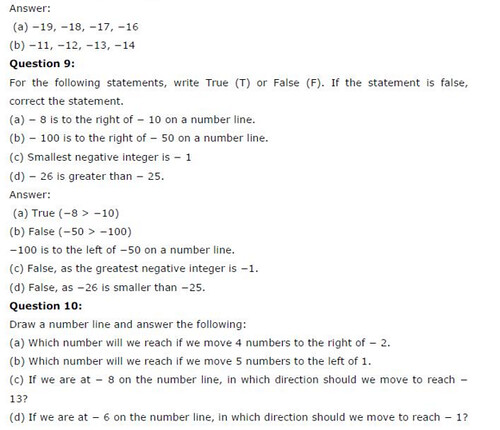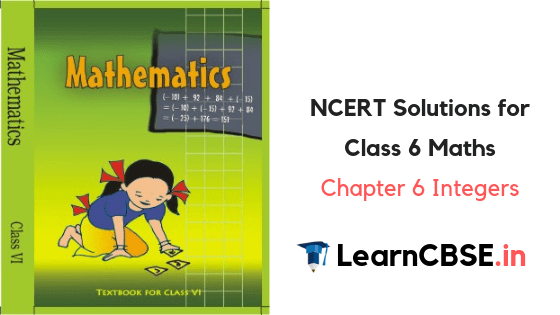## NCERT Solutions for Class 6 Maths Chapter 6 Integers Ex 6.1

Free download NCERT Solutions for Class 6 Maths Chapter 6 Exercise 6.1, Ex 6.2 and Ex 6.3 Integers PDF for CBSE 2020 Exams.

Topics and Sub Topics in Class 6 Maths Chapter 6 Integers

 Section Name Topic Name 6.1 Introduction 6.2 Integers 6.2.1 Representation of Integers on a Number Line 6.2.2 Ordering of Integers 6.3 Addition of Integers 6.3.1 Addition of Integers on a Number Line 6.4 Subtraction of Integers with the help of a Number Line

### Integers Class 6 Ex 6.1

Ex 6.1 Class 6 Maths Question 1.
Write opposites of the following:
(a) Increase in weight
(b) 30 km North
(c) 326 BC
(d) Loss of ₹700
(e) 100 m above sea level.
Solution:
(a) Decrease in weight
(b) 30 km South
(d) Profit of ₹700
(e)100 m below sea level.

Ex 6.1 Class 6 Maths Question 2.
Represent the following numbers as integers with appropriate signs.
(a) An aeroplane is flying at a height two thousand metre above the ground.
(b) A submarine is moving at a depth, eight hundred metre below the sea level.
(c) A deposit of rupees two hundred.
(d) Withdrawal of rupees seven hundred.
Solution:
(a) +2000 m
(b) -800 m
(c) + ₹200
(d) – ₹700

Ex 6.1 Class 6 Maths Question 3.
Represent the following numbers on a number line: ,
(a) +5
(b) -10
(c) ±8
(d) -1
(e) -6
Solution: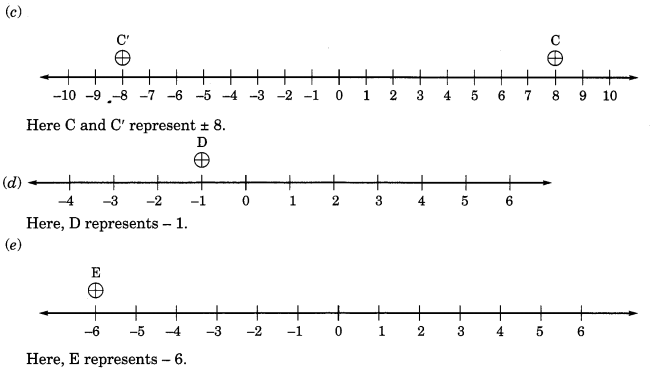Ex 6.1 Class 6 Maths Question 4.
Adjacent figure is a vertical number line, representing integers. Observe it and locate the following points: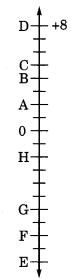(a) If point D is +8, then which point is -8?
(b) Is point G a negative integer or a positive integer?
(c) Write integers for points B and E.
(d) Which point marked on this number line has the least value?
(e) Arrange all the points in decreasing order of value.
Solution:
(a) F represents -8
(b) G is a negative integer.
(c) B represents +4 and E represents – 10
(d) E has the least value of -10.
(e) Decreasing order of all the points are: D, C, B, A, 0, H, G, F and E.

Ex 6.1 Class 6 Maths Question 5.
Following is the list of temperatures of five places in India on a particular day of the year.(a) Write the temperatures of these places in the form of integers in the blank column.
(b) Following is the number line representing the temperature in degree Celsius.Plot the name of the city against its temperature.
(c) Which is the coolest place?
(d) Write the names of the places where temperatures are above 10°C.
Solution: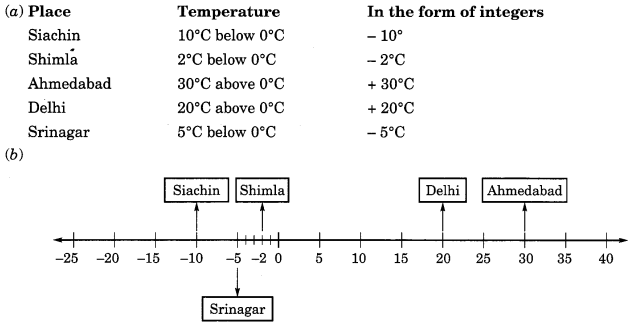(c) Siachin is the coolest place with -10°C temperature.
(d) (i) Delhi → 20°C

Ex 6.1 Class 6 Maths Question 6.
In each of the following pairs, which number is to the right of the other on the number line?
(a) 2, 9
(b) -3, -8
(c) 0, -1
(d) -11, 10
(e) -6, 6
(f) 1, -100
Solution:
(a) 9 is to the right of 2
(b) -3 is to the right of -8
(c) 0 is to the right of – 1
(d) 10 is to the right of -11
(e) 6 is to the right of -6
(f) 1 is to the right of -100.

Ex 6.1 Class 6 Maths Question 7.
Write all the integers between the given pairs (write them in the increasing order):
(a) 0 and -7
(b) -4 and 4
(c) -8 and -15
(d) -30 and -23
Solution:
(a) Integers between 0 and -7 are:
– 6, – 5, – 4, -3,-2,- 1.
(b) Integers between -4 and 4 are:
– 3, – 2, -1, .0, 1, 2, 3.
(c) Integers between -8 and -15 are:
-14,-13, -12,-11,-10,-9.
(d) Integers between -30 and -23 are:
– 29, – 28, – 27, – 26, – 25, – 24.

Ex 6.1 Class 6 Maths Question 8.
(a) Write four negative integers greater than -20.
(b) Write four negative integers less than – 10.
Solution:
(a) Four negative integers greater than – 20 are: – 19, – 18, – 17, – 16.
(b) Four negative integers less than – 10 are: – 11, – 12, – 13, – 14.

Ex 6.1 Class 6 Maths Question 9.
For the following statements, write True (T) or False (F).
If the statement is false, correct the statement.
(a) – 8 is to the right of – 10 on a number line.
(b) – 100 is to the right of- 50 on a number line.
(c) Smallest negative integer is – 1
(d) – 26 is greater than – 25.
Solution:
(a) True (T)
(b) False (F); Correction: -100 is to the left of -50 on a number line.
(c) False (F); Correction: There is no smallest negative integer.
(d) False (F); Correction: -26 is smaller than -25.

Ex 6.1 Class 6 Maths Question 10.
Draw a number line and answer the following:
(a) Which number will we reach if we move 4 numbers to the right of -2.
(b) Which number will we reach if we move 5 numbers to the left of 1.
(c) If we are at -8 on the number line, in which direction should we move to reach -13?
(d) If we are at -6 on the number line, in which direction should we move to reach -1?
Solution:### Integers Class 6 Ex 6.2

Ex 6.2 Class 6 Maths Question 1.
Using the number line write the integer which is:
(a) 3 more than 5
(b) 5 more than -5
(c) 6 less than 2
Solution:
(a) 3 more than 5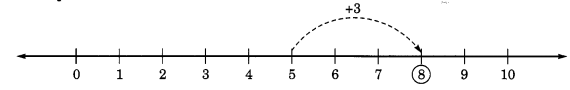Moving right 3 steps from 5, we reach at 8. Hence, 3 more than 5 = 8.

(b) 5 more than -5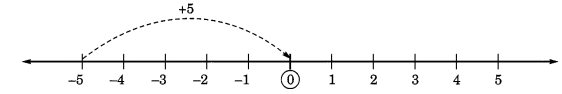Moving right 5 steps from -5 we reach at 0. Hence, 5 more than -5 = 0

(c) 6 less than 2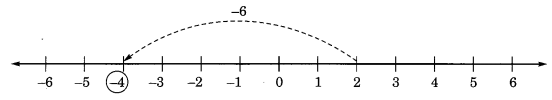Moving left 6 steps from 2, we reach at -4. Hence, 6 less than 2 = -4

(d) 3 less than -2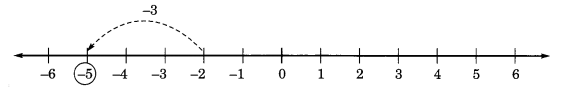Moving left 3 steps from -2, we reach at -5.

Ex 6.2 Class 6 Maths Question 2.
Use number line and add the following integers:
(a) 9 + (-6)
(b) 5 + (-11)
(c) (-1) + (-7)
(d) (- 5) + 10
(e) (-1) + (-2) + (-3)
Solution:
(a) 9 + (- 6)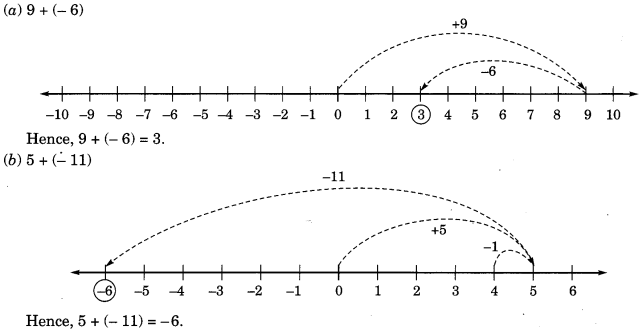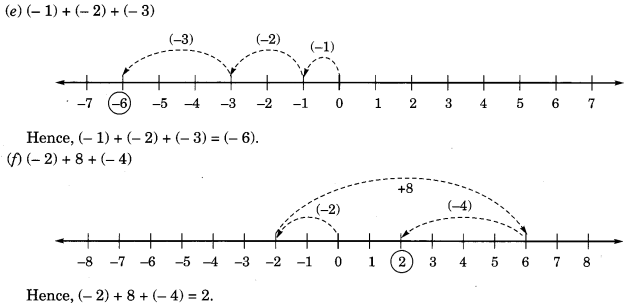Ex 6.2 Class 6 Maths Question 3.
(а) 11 + (-7)
(b) (-13) + (+18)
(c) (-10) + (+19)
(d) (-250) + (+150)
(e) (-380) + (-270)
(f) (-217) + (-100).
Solution:
(a) 11 + (-7) = 4 + (+7) + (-7)
[∵ (+7) + (-7) = 0]
= 4 + 0 = 4
Hence, 11 + (-7) = 4.

(b) (-13) + (+18) = (-13) + (+13) + (+5)
[∵ (-13) + (+13) = 0]
= 0 + (+ 5) = 5
Hence, (- 13) + (+ 18) = 5.

(c) (-10) + (+19) = (-10) + (+10) + (+9)
[∵ (-10) + (10) = 0] = 0 + (+9) = 9
Hence, (-10) + (19) = 9.

(d) (-250) + (+150) = (-100) + (-150) + (+150)
= (-100) + 0 = -100 [∵(-150) + (+150) = 0]
Hence, (-250) + (+150) = – 100.

(e) (-380) + (-270) = – [380 + 270] = (-650)
Hence, (-380) + (-270) = (-650).

(f) (-217) + (-100) = – [217 + 100] = -317

Ex 6.2 Class 6 Maths Question 4.
Find the sum of:
(a) 137 and -354
(b) -52 and 52 .
(d) -312, 39 and 192
(d) -50, -200 and 300
Solution:
(a) 137 and -354
(137) + ( -354) = (137) + (-137) + (-217) [∵ (137) + (-137) = 0]
= 0 + (-217) = (-217)

(b) -52 and 52
(-52) + (+52) = 0 [ ∵ (-a) + (+ a) = 0]

(c) -312, 39 and 192
(-312) + (+ 39) + (+192)
= (-231) + (- 81) + (+39) + (+192)
= (-231) + (-81) + (+ 231)
= (-231) + (+ 231) + (-81)
[∵ (-a) + (a) = 0]
= 0 + (- 81) = – 81

(d) – 50, -200 and 300
(-50) + (-200) + (+ 300)
= (-50) + (-200) + (+200) + (+100)
= (-50) + 0 + (+100)[∵ (-a) + (+ a) = 0]
= (-50) + (+100)
= (-50) + (+50) + (+ 50)
= 0 + (+ 50) = 50 [ ∵ (-a) + (+ a) = 0]

Ex 6.2 Class 6 Maths Question 5.
Find the sum of:
(a) (- 7) + (-9) + 4 + 16
(b) (37) + (-2) + (-65) + (-18)
Solution:
(a) (- 7) + (-9) + 4 + 16
= (-7) + (-9) + 4 + (+ 7) + (+ 9)
= (-7) + (+7) + (-9) + (+9) + 4
= 0 + 0 + 4 = 4 [∵ (-a) + (a) = 0]

(b) (37) + (-2) + (-65) + (-8)
= (+37) + (-75)
= (+37) + (-37) + (-38)
= 0 + (-38) = (-38) [∵ (-a) + (+a) = 0]

### Integers Class 6 Ex 6.3

Ex 6.3 Class 6 Maths Question 1.
Find:
(a) 35 – (20)
(b) 72 – (90)
(c) (-15) – (-18)
(d) (- 20) – (13)
(e) 23 – (-12)
(f) (-32) – (-40)
Solution:
(a) 35 – (20) = 15 + (20) – (20)
= 15 + 0 = 15 [(+a) + (-a) = 0]

(b) 72 – 90
72 – (72 + 18) = 72 – 72 – 18
= 0 – 18 = – 18 [a + (- a) = 0]

(c) (- 15) – (- 18)
= (- 15) + (additive inverse of – 18)
= (-15) + (18) = 3

(d) (- 20) – (13)
(- 20) – (13) = – [20 + 13] = – 33

(e) 23 – (- 12)
23 – (- 12) = 23 + (additive inverse of – 12)
= 23 + 12 = 35

(f) (- 32) – (- 40)
(- 32) + (additive inverse of – 40)
= (- 32) + 40 = 8

Ex 6.3 Class 6 Maths Question 2.
Fill in the blanks with >, < or = sign.
(a) (-3) + (-6) (-3) – (-6)
(b) (-21) – (-10) (- 31) + (-11)
(c) 45 – (-11) 57 + (-4)
(d) (-25) – (-42) (-42) – (-25)
Solution:
(a) (-3) + (-6) = – [3 + 6] = – 9 and (-3) – (-6) = (-3) + 6 = 3
Here, – 9 < 3
∴ (- 3) + (- 6) < (- 3) – (- 6)

(b) (-21) – (-10) = (-21) + 10 = -11 and (-31) + (-11) = – (31 + 11) = – 42
Here, -42 < -11 or -11 > -42 ∴ (-21) , -(-10) >(-31)+ (-11)

(c) 45 – (-11) = 45 + 11 = 56 and 57 + (-4) = 57 -4 = 53
Here, 56 >53
∴ 45 – (-11) > 57 + ( -4)

(d) (-25) – (-42) = -25 + 42 = 17
and (-42) – (-25) = -42 + 25 = -17
Here, 17 > -17
∴ (-25) – (-42) > (-42) – (-25).

Ex 6.3 Class 6 Maths Question 3.
Fill in the blanks.
(a) (-8) + …. = 0
(b) 13 + …. = 0
(c) 12 + (-12) = ….
(d) (-4) + …. = – 12
(e) …. -15 = – 10.
Solution:
(a) (-8) + (additive inverse of -8) = 0
= (-8) + (8) = 0
∴ Value of blank is 8

(b) 13 + (additive inverse of 13) = 0
= 13 + (-13) = 0
∴ Value of blank is – 13

(c) 12 + (-12) = 0 [∵ -12 is additive inverse of 12]
∴ The Value of blank is 0

(d) (-4) + (-8) = -[4 + 8] = -12
∴ Value of blank is -8.

(e) (+5) – 15 = -10
∴ Value of blank is +5.

Ex 6.3 Class 6 Maths Question 4.
Find :
(a) (-7) – 8 – (-25)
(b) (-13) + 32 – 8 – 1
(c) (-7) + (-8) + (-90)
(d) 50 – (-40) – (-2)
Solution:
(a) (-7) – 8 – (-25)
= (-7) – 8 + 25
[ ∵ Additive inverse of – 25 is 25]
= -7 + 17 = -7 + 7 +10
[∵ (-a) + (+a) = 0]
= 0 + 10 = 10.

(b) (-13) + 32 – 8 – 1
= (-13) + (13) + 19 – (8 + 1)
= 0 + 19 – 9
= 19 – 9 [∵ (-13) + (13) = 0]
= 10 + 9 – 9 = 10 + 0 = 10.
[(+9) – (+9) = 0]

(c) (-7) + (-8) + (-90) = – (7 + 8) + (-90)
= -15 + (-90)
= -(15 + 90)
= -105.

(d) 50 – (-40) – (-2)
= 50 – [- 40 – 2]
= 50 – (-42)
= 50 + 42
= 92.